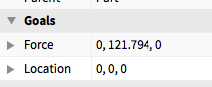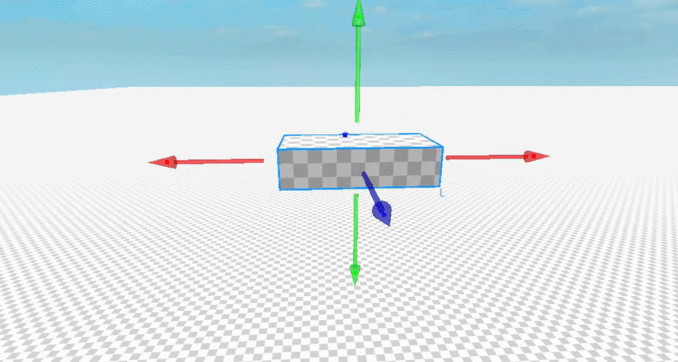# Hovering a bring using Bodythrust

i’ve been trying to figure out how i can hover a simple part(thruster) for something like a hover car, this is the math i’ve been using but sadly it doesn’t work.

`````` function gethoverinformation()
local ray = Ray.new(script.Parent.Position+Vector3.new(0,-10,0),((script.Parent.Position+Vector3.new(0,-10,0))-script.Parent.Position).unit*10)
return workspace:FindPartOnRay(ray,script.Parent)
end

local hvpower = script.Parent:GetMass()*196.2 -- our mass
local damping = 6 --damnping
local hoverheight = 5 --how hich we want to hover
local oldheight = 0 -- height we were on just a secodn ago

while true do
local Part,Pos = gethoverinformation() -- gets the part and hitposition below
local height = (Pos - script.Parent.Position).magnitude -- calculates the height between those 2 points
local deltaheight = (height-oldheight) -- how the height has changed
local power = (hoverheight-height-(deltaheight)*damping)*hvpower -- the math i am using
script.Parent.BodyThrust.Force = Vector3.new(0,power,0) -- trying to hover
coroutine.yield() -- once thats executed lets change the old height and start all overagain.
oldheight = height
end
``````

this is the formation i’ve been using. each part has the script and a body trust

anyone could figure out whats wrong with my math?

1 Like

The problem with your math is that you are simply calculating a force to bring it towards the hover height, so when it’s at the desired height you aren’t applying any more force. That means that it will just fall, until the force up is the same as the force down. You’ll end up hovering at some arbitrary point below the desired height (and possibly just land on the ground).

To have it hover at a given height, you will need to have it applying the same amount of force as gravity at that height, more below that height, and less above it.

If I were trying to solve this problem, I would first think: What force do I need to apply to counter gravity

Then I’d work out how to calculate this in my script. You already seem to have this part down.

``````Force = hvpower -- Mass * Gravity
``````

The easiest thing to do is then to just scale this based on the height. You need the scalar (multiplier) to be 1 when you are at the hover height, and it needs to be >1 if you are below it, and <1 when you are above it. That means that if you are below it, the force will beat gravity and pull it upwards. If you are above it and the force is less, gravity will win out and pull it back down.
So you would do something like this:

``````scalar = hoverheight / height -- Scalar multiplier for the force constant
Force = hvpower * scalar
``````

You can see that the scalar matches our criteria. If height is 10, and hoverheight is 5, then the scalar will be 0.5.
If the height is 5 and the hoverheight is 10, then the scalar will be 2.

This formula isn’t quite perfect, and a spring would work better, but it should be OK (especially with damping).

Damping is ofc also very simple, so I won’t bother explaining it. From your snippet you seem to understand it well.

Try this line:

``````local power = hoverheight / (height + deltaheight * damping) * hvpower
``````

Also, if I were you I would just use part.Velocity.Y instead of calculating deltaheight like that, but to each his own I guess.I haven’t tested this, so it might not work, but the theory should be sound.

3 Likes

well seem to work kinda, it doesn’t go in the negative this time. but rn the force is too low. and if i multiply it with the mass again it fly’s to no were

`````` function gethoverinformation()
local ray = Ray.new(script.Parent.Position+Vector3.new(0,-10,0),((script.Parent.Position+Vector3.new(0,-10,0))-script.Parent.Position).unit*10)
return workspace:FindPartOnRay(ray,script.Parent)
end

local hvpower = script.Parent:GetMass()*196.2
local damping = 6
local hoverheight = 5
local oldheight = 0

while true do
local Part,Pos = gethoverinformation()
local height = (Pos /script.Parent.Position).magnitude
local deltaheight = (height/oldheight)
local power = hoverheight / (height + deltaheight * damping) * hvpower
power = power * hvpower
script.Parent.BodyThrust.Force = Vector3.new(0,power,0)
coroutine.yield()
oldheight = height
end
``````what i came up with and works verry good is

``````local height = 10

while true do
wait()
local hit, pos = workspace.FindPartOnRay(workspace, Ray.new(script.Parent.Position, (script.Parent.CFrame * CFrame.Angles(-math.pi / 2, 0, 0)).lookVector * height* 2))
local dist = height * 2
if hit then
dist = (pos - script.Parent.Position).magnitude
end
local error = height - dist
script.Parent.BodyForce.force = Vector3.new(0, 500 * error, 0) - script.Parent.CFrame:vectorToObjectSpace(script.Parent.Velocity) * 30
end
``````

here you can see it in actionanyway thank you for your time and attempt to help me solve my math.4 Likes